# Statistics

Origin provides a number of options for performing general statistical analysis including: descriptive statistics, one-sample and two-sample hypothesis tests, and one-way and two-way analysis of variance (ANOVA). Also, several types of statistical charts are supported, including histograms and box charts

Advanced statistical analysis tools, such as repeated measures ANOVA, multivariate analysis, receiver operating characteristic (ROC) curves, power and sample size calculations, and nonparametric tests are available in OriginPro.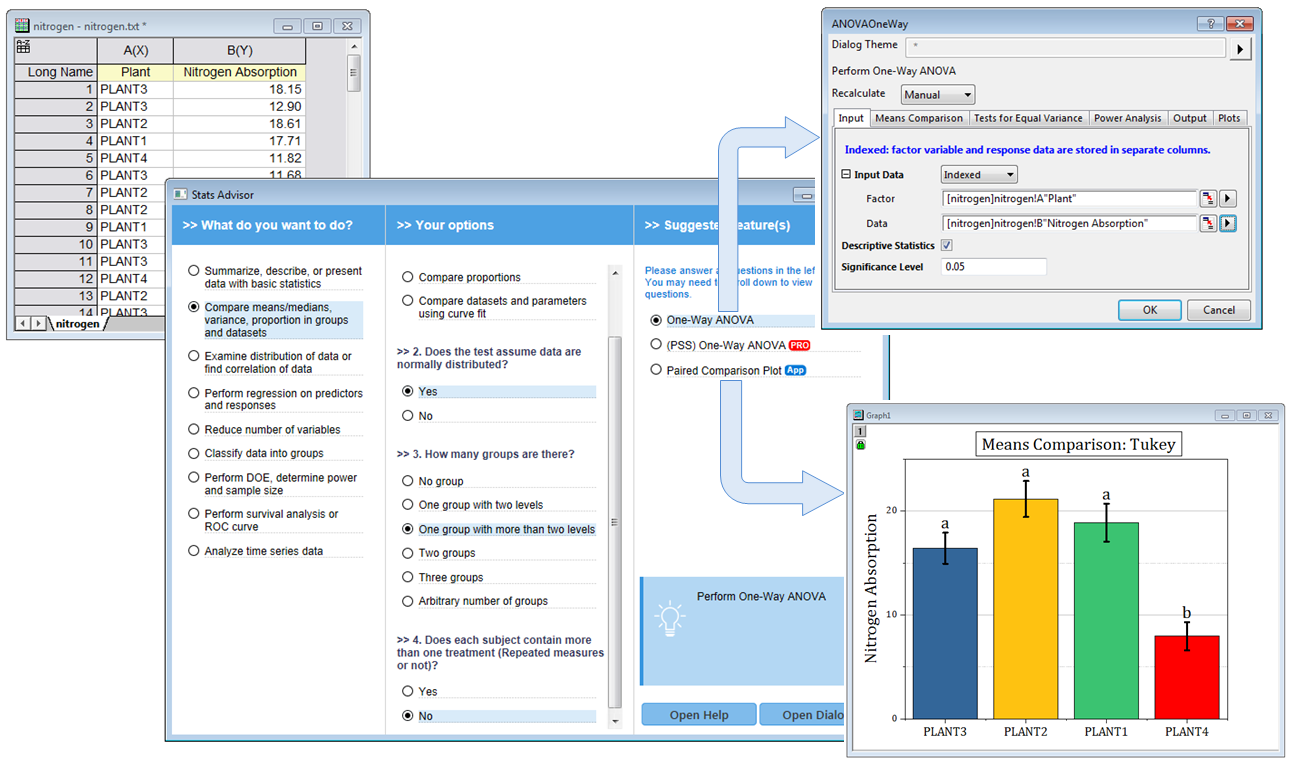The Stats Advisor App asks a series of questions and then suggests the appropriate tool or App to analyze your data.

## Descriptive Statistics

### Descriptive

The Statistics on Columns/Rows operation performs column-wise/row-wise descriptive statistics on selected worksheet data.

Statistics on Columns

Performs column-wise descriptive statistics on grouped or raw data.

Statistics on Rows

Performs row-wise descriptive statistics to generate statistics for rows in worksheet.

### Cross Tabulation PRO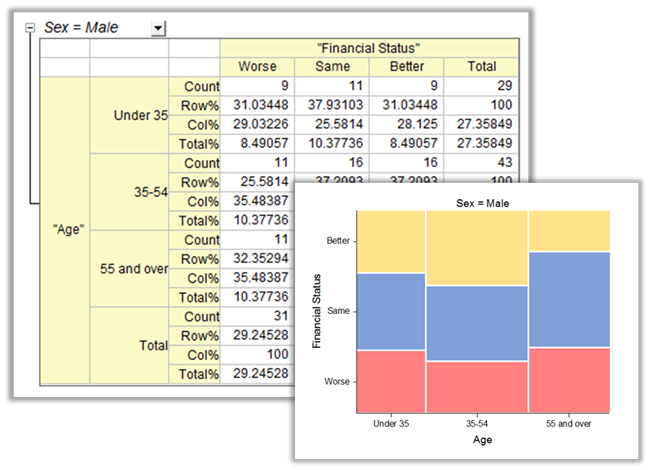Cross tabulation(also known as contingency table) is a table to reveal the frequency distribution of the variables. The mosaic graph can be plotted in the report.

Cross tabulation(also known as contingency table) is a table to reveal the frequency distribution of the variables. Analysis based on the table can determine whether there is a significant relationship, obtain the strength and direction of the relationship, and measure and test the agreement of matched-pairs data. It is widely used to analysis categorical data.

### Frequencies

Discrete Frequency

Discrete frequency analysis is one common method to analyze discrete variables. It counts the frequency of discrete data, including percentage and cumulative percentage.

Frequency Counts

The function computes the frequency counts for 1D data and help to produce histogram in desired way.

2D Frequency Count/Binning

A useful tool to compute the frequency counts and plot 2D histogram for 2D/bivariate data.tool is to measure the number of times a value is encountered that falls within each bin for a range of data. Then the results can be used to generate a histogram which allow more customization such as label on top of bars or having uneven bin size ect.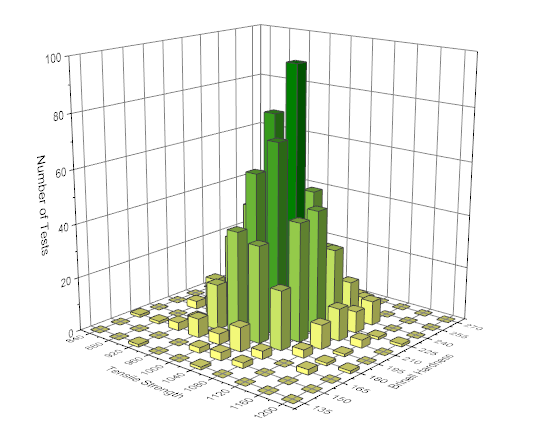The 2D Frequency Counts/Binning tool is similar to the Frequency Counts but for two-dimension variables. With the tool, we can generate 2D histogram to visually detect the distribution for 2D data.

### DistributionsUse Normality Test to determine whether data has been drawn from a normally distributed population (within some tolerance). Origin supports six methods for the normality test, Shapiro-Wilk, Kolmogorov-Smirnov, Lilliefors, Anderson-Darling, D'Agostino's K-Squared and Chen-Shapiro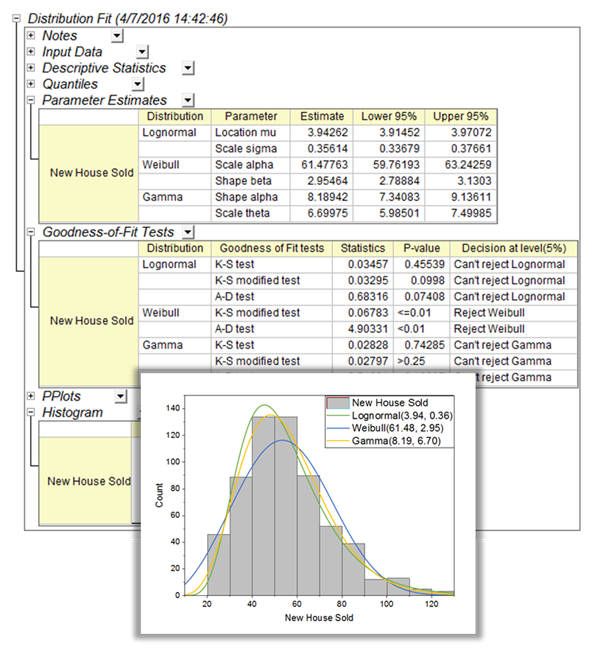The Distribution Fit tool helps users to examine the distribution of their data, and estimate parameters for the distribution

Normality Test

A normality test is used to determine whether sample data has been drawn from a normally distributed population (within some tolerance).

Six different normality tests are available in Origin:

• Shapiro-Wilk
• Kolmogorov-Smirnov
• Lilliefors
• Anderson-Darling
• D'Agostino's K-Squared
• Chen-Shapiro

Distribution Fit PRO

Knowing the distribution model of the data helps you to continue with the right analysis. or make estimation of your data. The Distribution Fit tool helps users to examine the distribution of their data, and estimate parameters for the distribution

### Correlation Coefficient PRO

Correlation Coefficient PRO

The correlation coefficient, also called the cross-correlation coefficient, is a measure of the strength of the relationship between pairs of variables. Origin provides both parametric and non-parametric measures of correlation.

• Pearson's r Correlation
• Spearman's Rank Order Correlation
• Kendall's tau Correlation

Partial Correlation Coefficient PRO

Partial correlation measures the linear relationship between two random variables, after excluding the effects of one or more control variables.The Partial Correlation tool measures the linear relationship between two random variables, after excluding the effects of one or more control variables.The image displays the Dialog of Correlation Coefficient tool in Origin. The tool supports three tests, Pearson's r Correlation, Spearman's Rank Order Correlation and Kendall's tau Correlation. And user can choose whether to flag the significant correlations in result

### Detecting Outliers

An outlier is an observation that is dramatically distant from the rest of the data. Origin provides two tools to help detecting the outliers.

 Grubbs Test Dixon's Q-test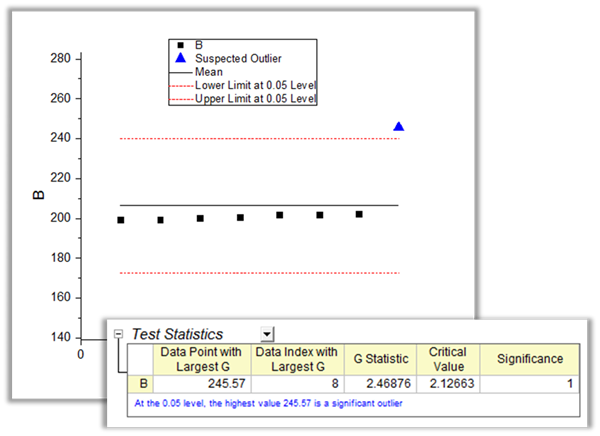Two tools in Origin can be used to detect outliers in data, Grubb's Test and the Dixon's Q-test. The outliers plot in the tools can help user to visually judge how the outlier is distant from other observations.

## ANOVA

Analysis of variance (ANOVA) is used to examine the differences between group means. In addition to determining that differences exist among the means, ANOVA tools in Origin provide multiple means comparisons in order to identify which particular means are different.

### One-Way, Two-Way and Three-Way ANOVA

One-way, two-way and three-way ANOVA consider a completely randomized design for an experiment.

One-Way ANOVA

One-way ANOVA compares three or more levels within one factor.

Two-Way ANOVA

Two-way ANOVA is useful to compare the effect of multiple levels of two factors. Two way ANOVA is an appropriate method to analyze the main effects of and interactions between two factors.

Three-Way ANOVA PRO

Three-way ANOVA ests for interaction effects between three independent variables on a continuous dependent variable (i.e., if a three-way interaction exists).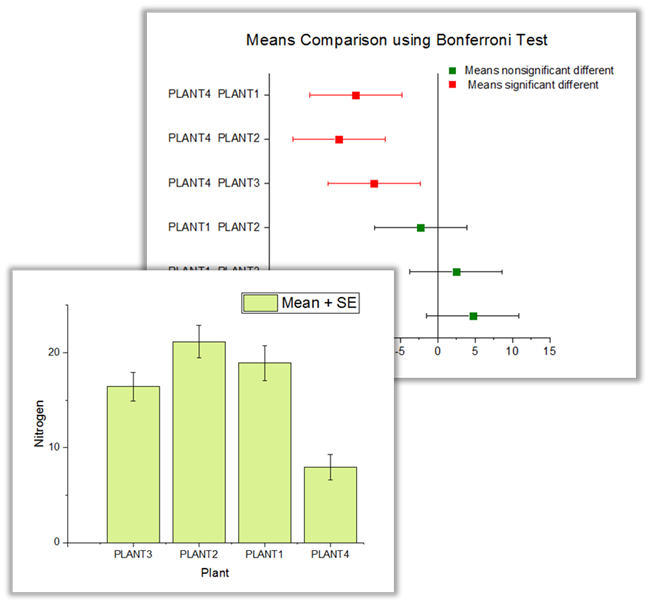The graph displays the Mean+SE plot and Means comparison plot in one-way anova. They are help to visually compare multiple groups, determine whether their means are different.The image displays results got in the one-way anova tool. The Overall ANOVA table reports a p-value that is smaller than 0.05, hence at least two of the four groups have significantly different means. There are also expandable Homogeneity of Variance Test and Means Comparisons table in result which helps to judge whether the groups have equal variance and provides pair-wise comparison.

### Repeated Measure ANOVA PRO

The repeated measures design is also known as a within-subject design. It has the same subjects performed under every condition.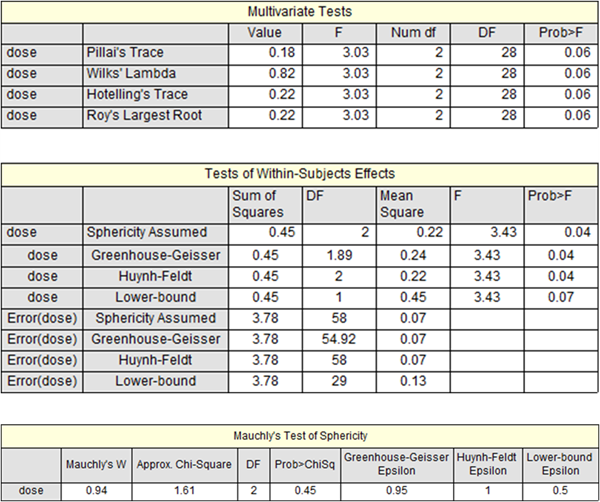The repeated measures ANOVA is used for comparing three or more means when all subjects are measured under a number of different conditions.

Repeated measure ANOVA tools in Origin consider three possible designs:

• One-way Repeated Measures PRO

ANOVA with one repeated-measures factor.

• Two-way Repeated Measures PRO

ANOVA with two repeated-measures factors.

• Two-way Mixed-Design PRO

The two-way mixed-design is also known as two way split-plot design (SPANOVA). It is ANOVA with one repeated-measures factor and one between-groups factor.

### Means Comparison / Post-hoc Tests

The mean comparison tests in ANOVA, also known as Post Hoc tests, are useful to perform additional comparisons of subsets of the means.

All four ANOVA tools in Origin, one and two-way ANOVA, one and two-way repeated measure ANOVA, provide seven means comparison tests:

• Tukey
• Bonferroni
• Dunn-Sidak
• Fisher LSD
• Sheff'
• Holm-Bonferroni
• Holm-SidakAn Origin Analysis Report Sheet, this one created by the One-way Repeated Measures ANOVA tool. The image shows two of the embedded graphs opened for further editing. Edit an embedded graph by double-clicking on the thumbnail image in the report. Once customizations are made, put the graphs back into the report and see your modifications.

## Parametric Hypothesis Tests

Parametric Hypothesis tests are frequently used to measure the quality of sample parameters or to test whether estimates on a given parameter are equal for two samples.

### T-Tests for Means

 One-Sample T-Test Pair-Sample T-Test Two-Sample T-Test

### T-Tests on Rows PRO

 Pair-Sample T-Test on Rows PRO Two-Sample T-Test on Rows PRO

### Variance Tests PRO

 One-Sample Test for Variance PRO Two-Sample Test for Variance PRO

### Proportion Tests PRO

 One-Sample Proportion Test PRO Two-Sample Proportion Test PRO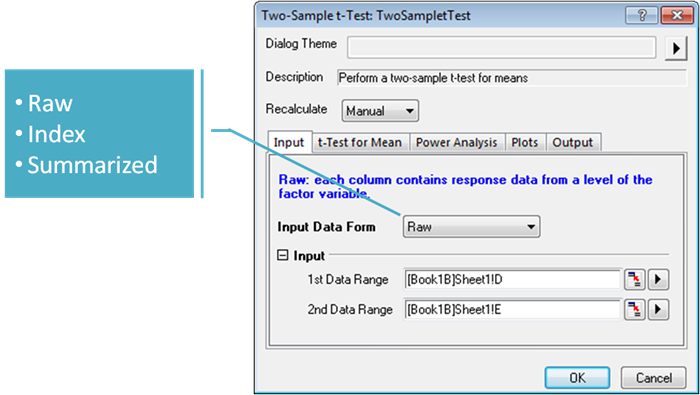Origin supports different input mode for hypothesis testing. User don't need to transform their data before using the tools.The example shows the results of two-sample t-test, a footnote is provided in the table(s) to help draw conclusions. Origin also support Welch's test for the case that variance is not equal.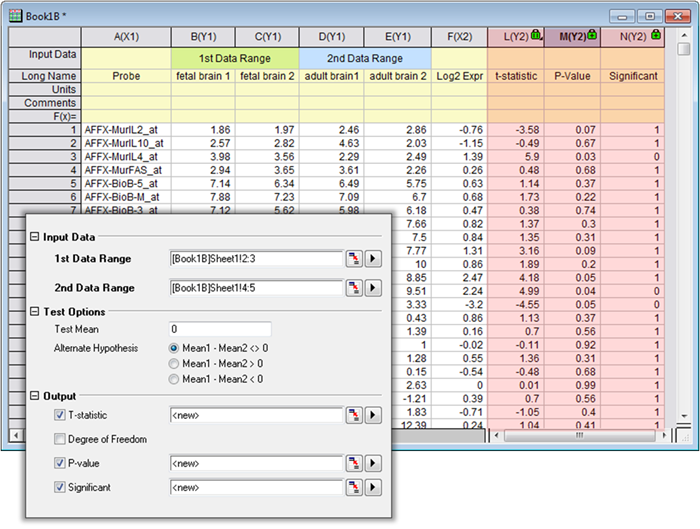The example shows dialog and results of two sample t-test on rows perform on gene data. The t-tests on rows tools in Origin enable user to compare data store in rows.

## Nonparametric Tests PRO

Nonparametric tests are useful for testing whether group means or medians are distributed the same across groups. In these types of tests, we rank (or place in order) each observation from our data set. Nonparametric tests are widely used when you do not know whether your data follows normal distribution, or you have confirmed that your data do not follow normal distribution. Meanwhile, hypothesis tests are parametric tests based on the assumption that the population follows a normal distribution with a set of parameters.

### One-Sample

Wilcoxon Signed Rank Test PRO

The One-Sample Wilcoxon Signed Rank Test is a nonparametric alternative to a one-sample t-test. The test determines whether the median of the sample is equal to some specified value. Data should be distributed symmetrically about the median.The One Sample Wilcoxon Signed Rank Test in Origin enable user to examine the population median relative to a specified value. In resuts, a footnote is provided in the table(s) to help to draw conclusions.

### Paired Samples

• Wilcoxon Signed Rank Test PRO

### Two Samples• Mann-Whitney PRO

• Kolmogorov-Smirnov Test PRO

### Multiple Independent Samples• Kruskal-Wallis ANOVA PRO

• Mood's Median Test PRO

### Multiple Related Samples

Friedman ANOVA PRO

Friedman ANOVA is a nonparametric alternative to the one way repeated measure ANOVA.

Friedman ANOVA can be used to compare dependent samples or observations that are repeated on the same subjects. Thus, the test is well-suited to randomized block designs.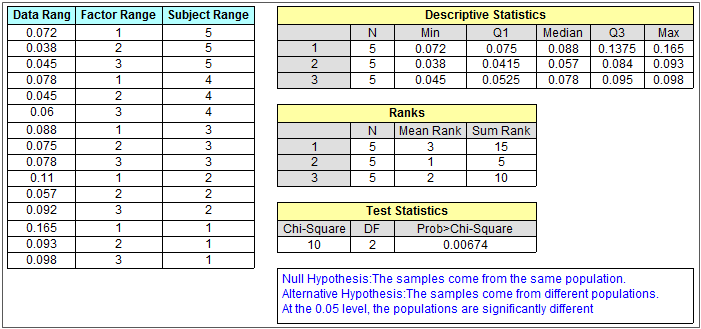The graphs shows the data and results of Friedman ANOVA. The tool in Origin can be used to compare three or more related samples. It is a nonparametric alternative to the one way repeated measure ANOVA.

## Multivariate Analysis PRO

Multivariate analysis is a set of techniques used to analyze data that corresponds to more than one variable. The main objective of this analysis is to study how the variables are related to one another, and how they work in combination to distinguish between multiple cases of observations.

### Principal Component Analysis PRO

Principal Component Analysis (PCA) is used to explain the variance-covariance structure of a set of variables through linear combinations of those variables. PCA is thus often used as a technique for reducing dimensionality.

### Cluster Analysis PRO

Cluster analysis is used to construct smaller groups with similar properties from a large set of heterogeneous data. This form of analysis is an effective way to discover relationships within a large number of variables or observations.

Hierarchical PRO

In this method, elements are grouped into successively larger clusters by some measures of similarity or distance.

K-means PRO

Use K-means clustering to classify observations through K number of clusters.

It is faster than Hierarchical but need user know the centroid of the observations, or at least the number of groups to be clustered.

### Discriminant Analysis PRO

Discriminant analysis is used to distinguish distinct sets of observations, and to allocate new observations to previously defined groups.

### Partial Least Squares Regression PRO

Partial Least Squares regression (PLS) is used for constructing predictive models when there are many highly collinear factors.

There are two primary reasons for using PLS:

• Prediction
PLS is most commonly used for constructing predictive model when the information contained in a large number of original variables and they are highly collinear.

• Interpretation
PLS can be used to discover important features of a large data set. It often reveals relationships that were previously unsuspected, thereby allowing interpretations of the data that may not ordinarily result from examination of the data.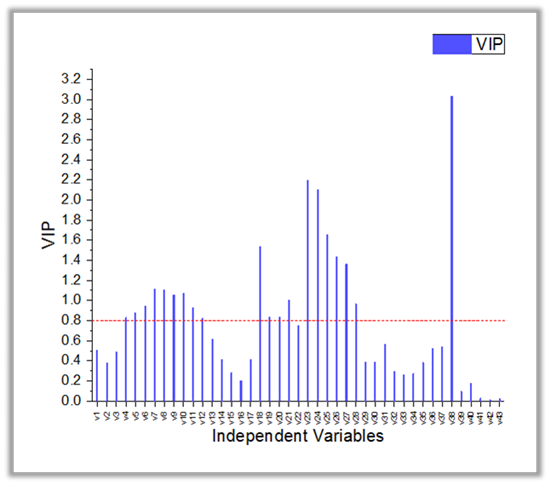The Partial Least Squares in Origin is used for constructing predictive models when there are many highly collinear factors. The Variable Importance Plot can help to judge the importance of each variable.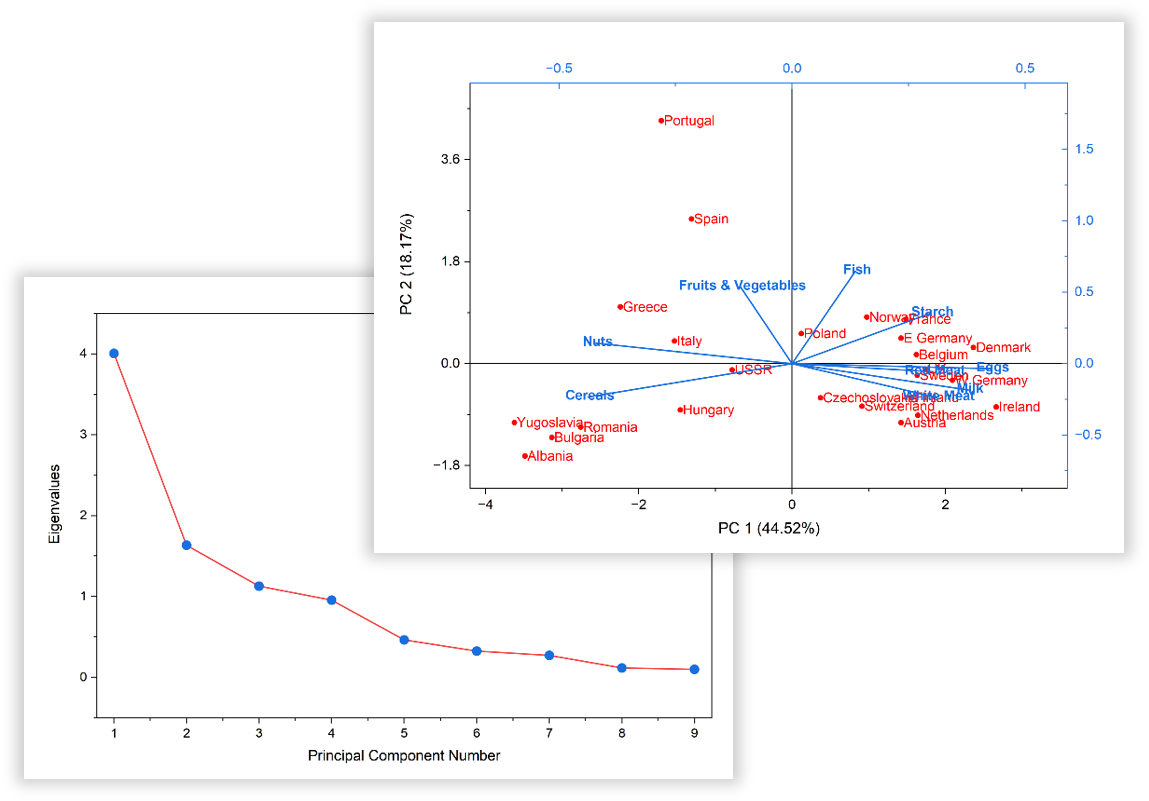The Principal Component Analysis (PCA) tool is used to explain the variance-covariance structure of a set of variables through linear combinations. The scree plot is a useful visual aid for determining an appropriate number of principal components. And the Loading and Score plot can be used for interpreting relations among observations and variables.A Dendrogram plot created by the Hierarchical Cluster Analysis tool, which can be used to list all samples and indicates at what level of similarity any two clusters were joinedA Canonical Score Plot created by the Discriminant Analysis tool in OriginPro. This plot can be used to classify observations across groups.

## Survival Analysis PRO

Survival Analysis is widely used in the biosciences to quantify survivorship in a population under study. OriginPro includes three widely used tests - the Kaplan-Meier (product-limit) Estimator, the Cox Proportional Hazards Model and the Weibull Fit.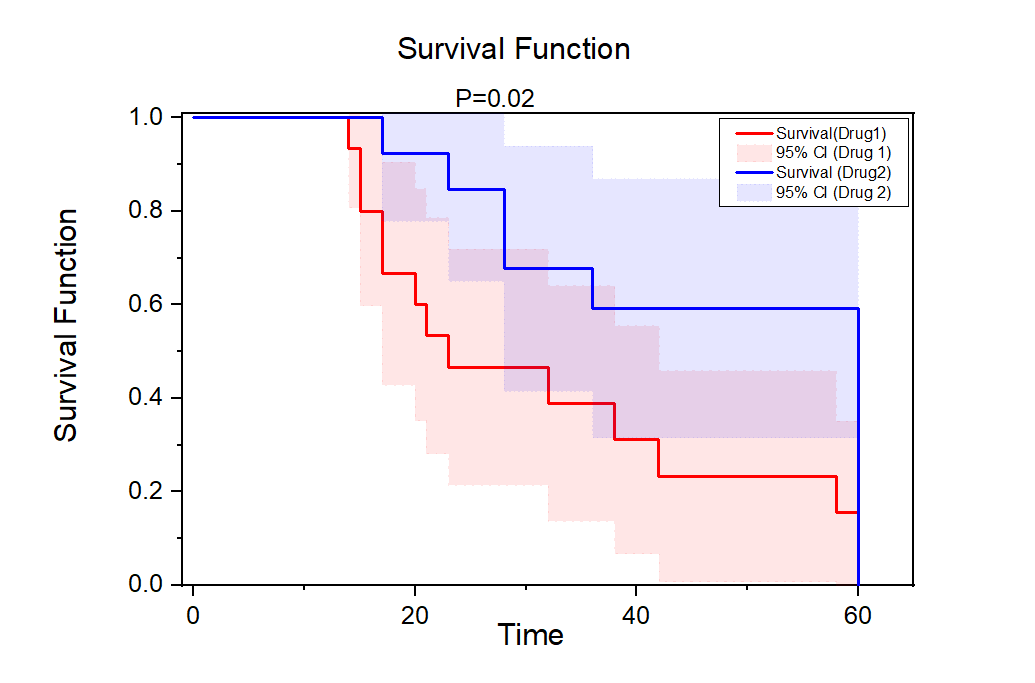The graph displays the survival function plot in Kaplan-Meier Estimator. A log-rank test is perform to compare the two survival function.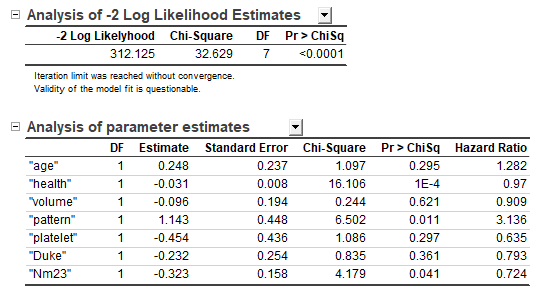The image displays a part of reports of the Cox Proportional Hazard Regression, which is a semi-parameter method to forecast changes in the hazard rate along with a variety of fixed covariates.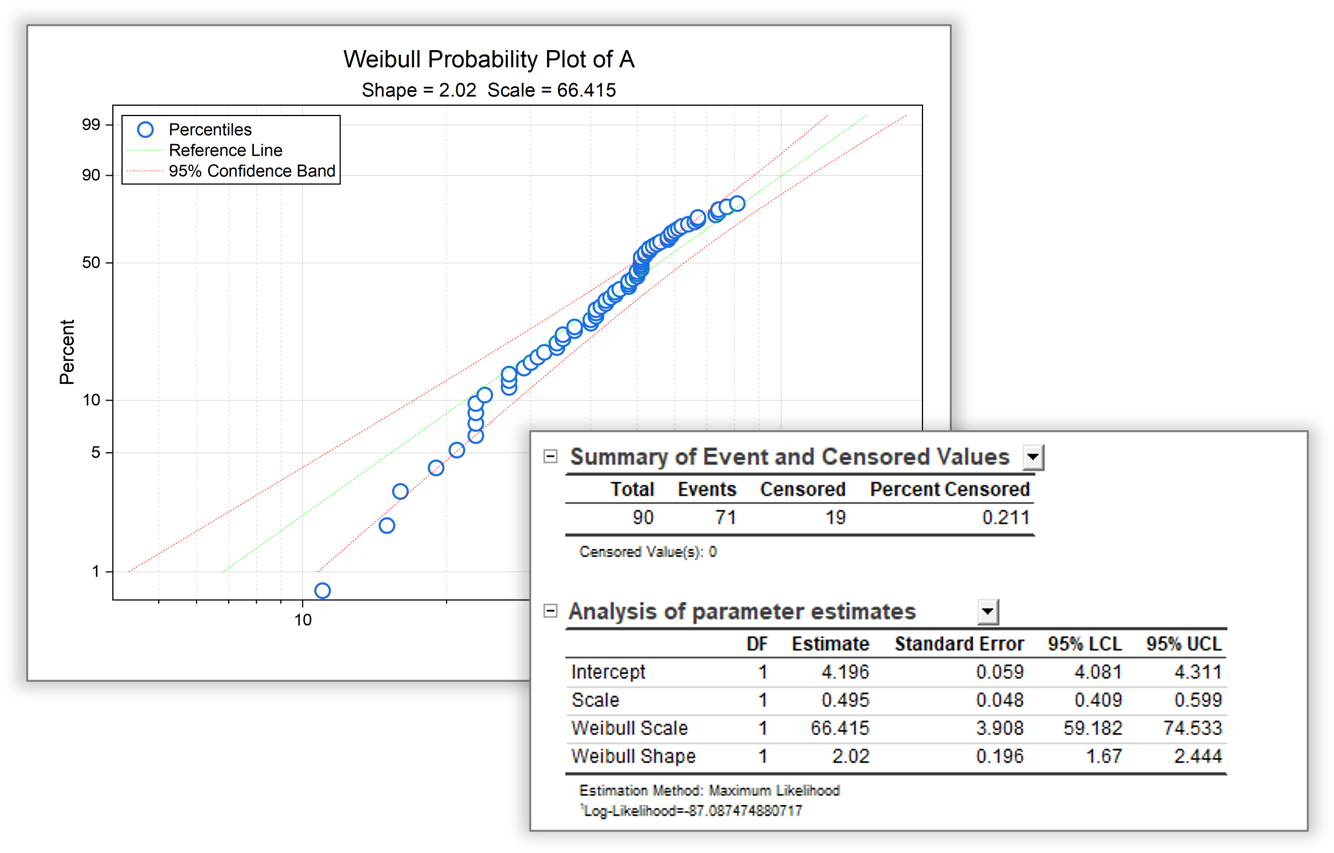The Weibull Fit is a parameter method to analyze the relationship between the survival function and the failure time. User can see the parameter estimation of the Weibull model from the result table and visually decide whether the data are drop from Weibull distribution from the Weibull Probability Plot

### Kaplan-Meier Estimator PRO

Kaplan-Meier Estimator, a non-parametric estimator, uses product-limit methods to estimate the survival function from lifetime data.

In addition to estimating the survival functions, Kaplan-Meier Estimator in Origin provides three other methods to compare the survival function between two samples:

• Log Rank
• Breslow
• Tarone-Ware

### Cox Proportional Hazard Model PRO

The proportional hazards model, also called Cox model, is a classical semi-parameter method. It relates the time of an event, usually death or failure, to a number of explanatory variables known as covariates.

### Weibull Fit PRO

Weibull fit is a parameter method to analyze the relationship between the survival function and the failure time. We suppose that the survival function follows a Weibull distribution and fit the model with a maximum likelihood estimation.

## Power and Sample Size PRO

Power and Sample Size analysis is useful for researchers to design their experiments. It can compute the power of the experiment for a given sample size, and can also compute the required sample size for given power values.

The following testings are available:

 (PSS)One-Sample t-Test (PSS)Two-Sample t-Test (PSS)Paired-Sample t-Test (PSS)One-Proportion Test (PSS)Two-Proportion Test (PSS)One-Variance Test (PSS)Two-Variance Test (PSS)One-Way ANOVA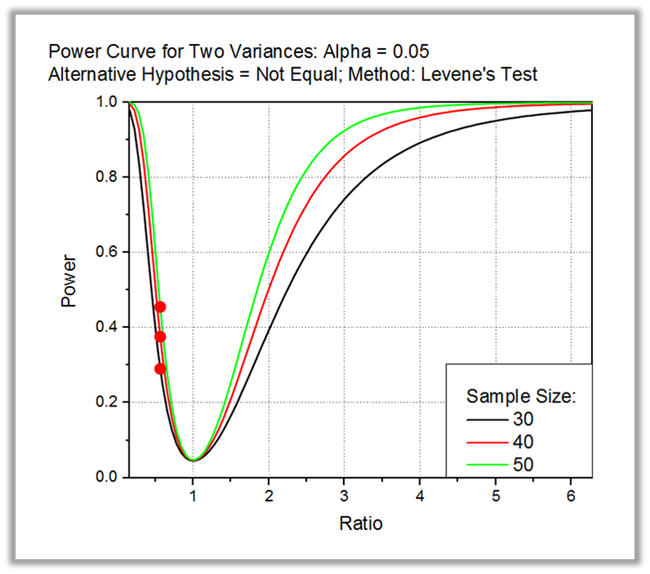Power and Sample Size Analysis includes both sample size analysis and power analysis. The sample size analysis is used to determine whether an experiment is likely to yield useful information with a given sample size, Conversely, power analysis can be useful in determining the minimum sample size needed to produce a statistically significant experiment. The graph displays the the power curve for the two variances test.

## ROC Curve PROThe ROC Curve analysis can be used to test a diagnostic to determine if an incident had occurred, or compare the accuracy of two methods that are used to discriminate diseased cases versus healthy cases.

ROC (Receiver Operating Characteristic) curve analysis is mainly used for diagnostic studies in Clinical Chemistry, Pharmacology and Physiology. It has been widely accepted as the standard tool for describing and comparing the accuracy of diagnostic tests.

For example, you can use ROC Curve analysis to test a diagnostic to determine if an incident had occurred, or compare the accuracy of two methods that are used to discriminate diseased cases versus healthy cases.

## Apps